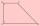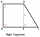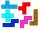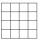# Square (second power, quadratic) + trapezoid - math problems

#### Number of problems found: 32

• TrapezoidAre diagonals in a rectangular trapezoid perpendicular and bisect the angles?
• Trapezoid ABCD v2Trapezoid ABCD has length of bases in ratio 3:10. The area of riangle ACD is 825 dm2. What is the area of trapezoid ABCD?
• A trapezoidA trapezoid 75 ft wide on top 85 ft on the bottom, the height is 120 ft. What is its area in the square yds?
• ISO trapezoid v2bases of Isosceles trapezoid measured 16 cm and 4 cm and its perimeter is 47 cm. What is the are of a trapezoid?
• The sides 2The sides of a trapezoid are in the ratio 2:5:8:5. The trapezoid’s area is 245. Find the height and the perimeter of the trapezoid.
• Trapezoid 15Area of trapezoid is 266. What value is x if bases b1 is 2x-3, b2 is 2x+1 and height h is x+4
• Right trapezoidThe right trapezoid has bases 3.2 cm and 62 mm long. The shorter leg has a length 0.25 dm. Calculate the lengths of the diagonals and the second leg.
• Trapezium zoomHow many times increase area of trapezoid if all sides and altitude increase 5 times?
• TrapezoidArea of trapezoid is 135 cm2. Sides a, c and height h are in a ratio 6:4:3. How long are a,c and h? Make calculation...
• Calculate 7Calculate the height of the trapezoid ABCD, where coordinates of vertices are: A[2, 1], B[8, 5], C[5, 5] and D[2, 3]
• EstateEstate shaped rectangular trapezoid has bases long 34 m , 63 m and perpendicular arm 37 m. Calculate how long is its fence.
• GardenTrapezoid garden has parallel sides 19 m and 24 m. Its area is 193.5 square meters. What is the width of the garden?
• LegDetermine the area of a trapezoid with bases 71 and 42 and height is 4 shorter than the its leg.
• SquareSquare JKLM has sides of length 24 cm. Point S is the center of LM. Calculate the area of the quadrant JKSM in cm2.
• The ditchDitch with a cross-section of an isosceles trapezoid with bases 2m and 6m are deep 1.5m. How long is the slope of the ditch?
• IS trapezoidCalculate the length of diagonal u and height v of isosceles trapezoid ABCD, whose bases have lengths a = |AB| = 37 cm, c = |CD| = 29 cm and legs b = d = |BC| = |AD| = 28 cm.
• Isosceles trapezoidThe old father decided to change the top plate of an isosceles-like trapezoid with the basic dimensions of 120 cm and 60 cm, and the shoulder is 50 centimeters long. How much does it pay for a new plate and a square meter worth 17 euros?
• Rectangular trapezoidThe ABCD rectangular trapezoid with the AB and CD bases is divided by the diagonal AC into two equilateral rectangular triangles. The length of the diagonal AC is 62cm. Calculate trapezium area in cm square and calculate how many differs perimeters of the
• Square prismCalculate the volume of a foursided prism 2 dm high, the base is a trapezoid with bases 12 cm, 6 cm, height of 4 cm and 5 cm long arms.Road embankment has a cross section shape of an isosceles trapezoid with bases 5 m and 7 m, and 2 m long leg. How many cubic meters of soil is in embankment length of 1474 meters?